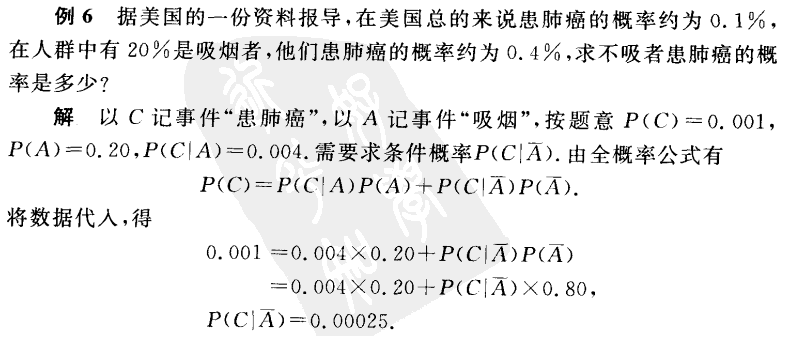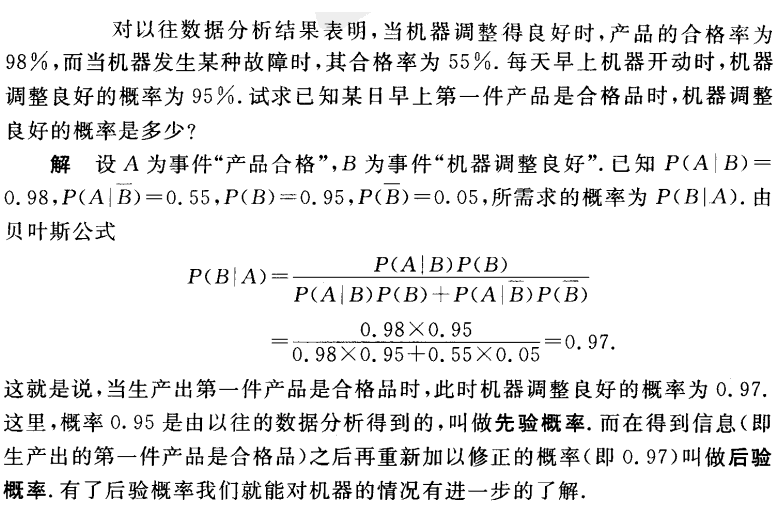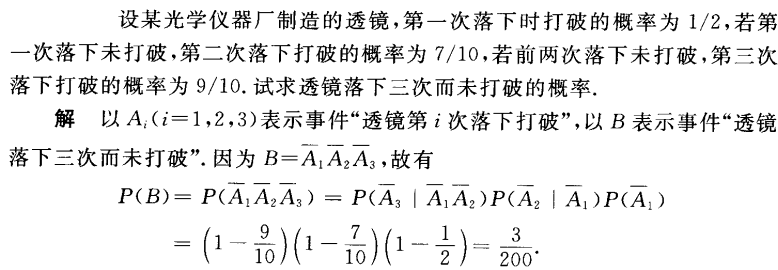• 本节为概率论与数理统计复习笔记的第二节，随机事件与概率(2)，主要包括：加法公式、减法公式、条件概率公式、乘法公式、全概率公式、贝叶斯公式以及两道例题。

本节为概率论与数理统计复习笔记的第二节，随机事件与概率(2)，主要包括：加法公式、减法公式、条件概率公式、乘法公式、全概率公式、贝叶斯公式以及两道例题。

1.常用的求概率公式
1.加法公式
$P(A\cup B)=P(A)+P(B)-P(AB)\\\ \\ P(A\cup B \cup C)=\\P(A)+P(B)+P(C)\\-P(AB)-P(AC)-P(BC)+P(ABC)$
2.减法公式
$P(A-B)=P(A)-P(AB)=P(A\bar B)$
3.条件概率公式
已知事件A发生的条件下，事件B发生的概率：
$P(B|A)=\frac{P(AB)}{P(A)}$
引申一下还有：
$P(\bar B|A)=1-P(B|A)\\\ \\ P(B-C|A)=P(B|A)-P(BC|A)$
4.乘法公式
$P(AB)=P(A)P(B|A)=P(B)P(A|B)\\\ \\ P(ABC)=P(A)P(B|A)P(C|AB)$
5.全概率公式（全集分解公式）
若$\cup_{i=1}^n A_i=\Omega，A_iA_j=\empty（i\neq j;i,j=1,...,n）$，则对任一事件B有$B=\cup_{i=1}^n A_iB，P(B)=\sum_{i=1}^nP(A_i)P(B|A_i)$。
6.贝叶斯公式（逆概公式）
如果$\cup_{i=1}^n A_i=\Omega，A_iA_j=\empty（i\neq j;i,j=1,...,n），P(A_i)>0$，则对任一事件$B$，只要$P(B)>0$，即有$P(A_j|B)=\frac{P(A_j)P(B|A_j)}{\Sigma_{i=1}^nP(A_i)P(B|A_i)}$。
2. 两道例题
eg1.设有两批数量相同的零件，已知有一批产品全部合格，另一批产品有25%不合格。从两批产品中任取已知，经检验是正品，放回原处，并在原处所在批次再取一只，试求这只产品是次品的概率。
解：设事件$H_i(i=1,2)$为“第一次从第i批产品中抽取”，事件$A$为取正品，则$P(H_1)=P(H_2)=\frac12，P(A|H_1)=1$，$P(A|H_2)=\frac34$。
则有$P(A)=P(H_1)P(A|H_1)+P(H_2)P(A|H_2)=\frac78$（全概率公式）；
从而（贝叶斯）：
$P(H_1|A)=\frac{P(H_1)P(A|H_1)}{P(A)}=\frac47\\\ \\ P(H_2|A)=\frac{P(H_2)P(A|H_2)}{P(A)}=\frac37$(当第一次取正品之后，概率就发生了变化，不是1/2了)
设$C_i(i=1,2)$表示“第二次从第i批产品中抽取”，则有：
$P(\bar A)=P(C_1)P(\bar A|C_1)+P(C_2)P(\bar A|C_2)\\\ \\=\frac47 \times 0+\frac37 \times \frac14=\frac3{28}$
eg2.设有两箱零件，第一箱内装50件，其中10件是一等品；第二箱内装30件，其中18件是一等品。
求：
（1）先去除的零件是一等品的概率$p$；
（2）在先取出的是一等品的条件下，后取出的零件仍然是一等品的概率$q$。
解：记$A$={从第一箱中取}，$B_1$={先取出的是一等品}，$B_2$={后取出的是一等品}，则有：
$P(A)=P(\bar A)=\frac12，P(B_1|A)=\frac15，P(B_1|\bar A)=\frac35$
$P(B_1B_2|A)=\frac{10}{50}\times \frac9{49}，P(B_1B_2|\bar A)=\frac{18}{30}\times \frac{17}{29}$
（1）$p=P(A)P(B_1|A)+P(\bar A)P(B_1|\bar A)=\frac25$
（2）$P(B_1B_2)=P(A)P(B_1B_2|A)+P(\bar A)P(B_1B_2|\bar A)=\frac{276}{1421}$，
则$q=P(B_2|B_1)=\frac{P(B_1B_2)}{P(B_1)}=\frac{690}{1421}$。

欢迎扫描二维码关注微信公众号 深度学习与数学    [每天获取免费的大数据、AI等相关的学习资源、经典和最新的深度学习相关的论文研读，算法和其他互联网技能的学习，概率论、线性代数等高等数学知识的回顾]展开全文• 概率公式3.Bayes 公式4.乘法公式4.独立性1.事件的独立性2.独立重复试验3.重要公式结论 1.概率基本概念 1.随机试验、样本空间、随机事件 随机试验：扔硬币、掷骰子 试验 描述 E1E1E1 抛掷一...
概率基础：随机事件和概率1.概率基本概念1.随机试验、样本空间、随机事件2.概率2.事件的关系与运算1.事件关系2.运算律3.完全事件组3.概率的基本公式1.条件概率2.全概率公式3.Bayes 公式4.乘法公式4.独立性1.事件的独立性2.独立重复试验3.重要公式与结论
1.概率基本概念
1.随机试验、样本空间、随机事件
随机试验：扔硬币、掷骰子

试验
描述

$E1$
抛掷一枚硬币，观察正面$H$、反面$T$出现的情况

$E2$
将一枚硬币抛掷三次，观察正面$H$、反面$T$出现的情况

$E3$
将一枚硬币抛掷三次，观察正面$H$的次数

$E4$
抛掷一枚硬币，观察出现的点数

样本空间：随机试验E的所有可能结果构成的集合称为E的样本空间

样本空间
描述

$S1$
$\{H,T\}$

$S1$
$\{HHH,HHT,HTH,THH,HTT,THT,TTH,TTT\}$

$S1$
$\{0,1,2,3\}$

$S1$
$\{1,2,3,4,5,6\}$

随机事件：试验$E$的样本空间$S$的任意一个子集称为$E$的随机事件，简称事件.
2.概率
定义  设$E$是随机试验，$S$是它的样本空间，对于$E$的每一事件$A$赋予一个实数，记为$P(A)$，称为事件$A$的概率，如果集合函数$P(\centerdot )$满足下列条件：

非负性：对于每一个事件$A$，有$P(A)\geq0$；
规范性：对于必然事件$S$，有$P(S)=1$；
可列可加性：设${\mathrm A}_1,{\mathrm A}_2,\cdots$是两两互不相容的事件，即对于${\mathrm A}_i{\mathrm A}_{\mathrm j}=\varnothing,i\neq j,i,j,=1,2,\cdots$有$P\left(A_1\cup A_2\cup\cdots\right)=P\left(A_1\right)+P\left(A_2\right)+\cdots$

2.事件的关系与运算
1.事件关系
(1) 子事件：$A \subset B$，若$A$发生，则$B$发生。
(2) 相等事件：$A = B$，即$A \subset B$，且$B \subset A$ 。
(3) 和事件：$A\bigcup B$（或$A + B$），$A$与$B$中至少有一个发生。
(4) 差事件：$A - B$，$A$发生但$B$不发生。
(5) 积事件：$A\bigcap B$（或${AB}$），$A$与$B$同时发生。
(6) 互斥事件（互不相容）：$A\bigcap B$=$\varnothing$。
(7) 互逆事件（对立事件）：$A\bigcap B=\varnothing ,A\bigcup B=\Omega ,A=\bar{B},B=\bar{A}$
2.运算律
(1) 交换律：$A\bigcup B=B\bigcup A,A\bigcap B=B\bigcap A$
(2) 结合律：$(A\bigcup B)\bigcup C=A\bigcup (B\bigcup C)$
(3) 分配律：$(A\bigcap B)\bigcap C=A\bigcap (B\bigcap C)$
(4)德摩根律：$\overline{A\bigcup B}=\bar{A}\bigcap \bar{B}$ $\overline{A\bigcap B}=\bar{A}\bigcup \bar{B}$
3.完全事件组
${{A}_{1}}{{A}_{2}}\cdots {{A}_{n}}$两两互斥，且和事件为必然事件，即$A_i\cap A_i=\varnothing，i\neq j,\bigcup_{i=1}^n=\Omega$
3.概率的基本公式
1.条件概率
$P(B|A)=\frac{P(AB)}{P(A)}$,表示$A$发生的条件下，$B$发生的概率。
2.全概率公式
$P\left( A \right) =\sum\limits_{i=1}^n{P\left( A|B_i \right) P\left( B_i \right) =P\left( A|B_1 \right) P\left( B_1 \right) +P\left( A|B_2 \right) P\left( B_2 \right) +\cdots +,B_iB_j}=\varnothing ,i\ne j,\underset{i=1}{\overset{n}{\bigcup{}}}\,B_i=\Omega$3.Bayes 公式
$P({{B}_{j}}|A)=\frac{P(A|{{B}_{j}})P({{B}_{j}})}{\sum\limits_{i=1}^{n}{P(A|{{B}_{i}})P({{B}_{i}})}},j=1,2,\cdots ,n$
注：上述公式中事件${{B}_{i}}$的个数可为可列个。4.乘法公式
$P({{A}_{1}}{{A}_{2}})=P({{A}_{1}})P({{A}_{2}}|{{A}_{1}})=P({{A}_{2}})P({{A}_{1}}|{{A}_{2}})$
$P({{A}_{1}}{{A}_{2}}\cdots {{A}_{n}})=P({{A}_{1}})P({{A}_{2}}|{{A}_{1}})P({{A}_{3}}|{{A}_{1}}{{A}_{2}})\cdots P({{A}_{n}}|{{A}_{1}}{{A}_{2}}\cdots {{A}_{n-1}})$4.独立性
1.事件的独立性
(1)$A$与$B$相互独立  $\Leftrightarrow P(AB)=P(A)P(B)$
(2)$A$，$B$，$C$两两独立
$\Leftrightarrow P(AB)=P(A)P(B)$;$P(BC)=P(B)P(C)$ ;$P(AC)=P(A)P(C)$;
(3)$A$，$B$，$C$相互独立
$\Leftrightarrow P(AB)=P(A)P(B)$; $P(BC)=P(B)P(C)$ ;
$P(AC)=P(A)P(C)$ ; $P(ABC)=P(A)P(B)P(C)$
2.独立重复试验
将某试验独立重复$n$次，若每次实验中事件 A 发生的概率为$p$，则$n$次试验中$A$发生$k$次的概率为：
$P(X=k)=C_{n}^{k}{{p}^{k}}{{(1-p)}^{n-k}}$
3.重要公式与结论
$(1)P(\bar{A})=1-P(A)$
$(2)P(A\bigcup B)=P(A)+P(B)-P(AB)$
$P(A\bigcup B\bigcup C)=P(A)+P(B)+P(C)-P(AB)-P(BC)-P(AC)+P(ABC)$
$(3)P(A-B)=P(A)-P(AB)$
$(4)P(A\bar{B})=P(A)-P(AB),P(A)=P(AB)+P(A\bar{B}),$
$P(A\bigcup B)=P(A)+P(\bar{A}B)=P(AB)+P(A\bar{B})+P(\bar{A}B)$
(5)条件概率$P(\centerdot |B)$满足概率的所有性质，
例如：. $P({{\bar{A}}_{1}}|B)=1-P({{A}_{1}}|B)$
$P({{A}_{1}}\bigcup {{A}_{2}}|B)=P({{A}_{1}}|B)+P({{A}_{2}}|B)-P({{A}_{1}}{{A}_{2}}|B)$
$P({{A}_{1}}{{A}_{2}}|B)=P({{A}_{1}}|B)P({{A}_{2}}|{{A}_{1}}B)$
(6)若${{A}_{1}},{{A}_{2}},\cdots ,{{A}_{n}}$相互独立，则$P(\bigcap\limits_{i=1}^{n}{{{A}_{i}}})=\prod\limits_{i=1}^{n}{P({{A}_{i}})},$
$P(\bigcup\limits_{i=1}^{n}{{{A}_{i}}})=\prod\limits_{i=1}^{n}{(1-P({{A}_{i}}))}$
(7)互斥、互逆与独立性之间的关系：
$A$与$B$互逆$\Rightarrow$ $A$与$B$互斥，但反之不成立，$A$与$B$互斥（或互逆）且均非零概率事件$\Rightarrow$$A$与$B$不独立.
(8)若${{A}_{1}},{{A}_{2}},\cdots ,{{A}_{m}},{{B}_{1}},{{B}_{2}},\cdots ,{{B}_{n}}$相互独立，则$f({{A}_{1}},{{A}_{2}},\cdots ,{{A}_{m}})$与$g({{B}_{1}},{{B}_{2}},\cdots ,{{B}_{n}})$也相互独立，其中$f(\centerdot ),g(\centerdot )$分别表示对相应事件做任意事件运算后所得的事件，另外，概率为 1（或 0）的事件与任何事件相互独立.


展开全文• 1 重要概念与公式： 1.1 样本空间——$$\Omega$$（全集），其基本元素$$\omega_i$$叫样本点 1.2 事件——样本空间的子集$$A、B、C$$… $$\Phi$$——不可能事件 $$\Omega$$——必然事件 1.3 完备事件组 $$\... 1 重要概念与公式： 1.1 样本空间——\(\Omega$$（全集），其基本元素$$\omega_i$$叫样本点

1.2 事件——样本空间的子集$$A、B、C$$…

$$\Phi$$——不可能事件

$$\Omega$$——必然事件

1.3 完备事件组

$$\cup_i{A_i}=\Omega$$

$${A_i}\cap{A_j}=\Phi (两两互斥)(交集可省略符号, 写成A_iA_j=\Phi), i\neq{j}$$

1.4 运算、关系

——略，可看集合论中的运算、关系

2 古典概型

若$$\Omega$$中有有限个、等可能的样本点，称为古典概型

$$P(A)={{N_A}\over{N_\Omega}}$$

3 几何概型

若$$\Omega$$是一个可度量的集合区域，且样本点落入$$\Omega$$中的某一可度量子区域A的可能性大小与A的几何度量成正比，而与A的位置和形状无关，称为几何概型

$$P(A)={{M_A}\over{M_\Omega}}$$

$$M代表度量measure，可以是面积S，长度L等$$

4 重要公式

4.1 对立事件：

$$P(A)=1-P(\bar{A})$$

4.2 事件减法：

$$P(A-B)=P(A)-P(AB)$$

4.3 事件加法：

$$P(A+B)=P(A)+P(B)-P(AB)$$

$$P(A+B+C)=P(A)+P(B)+P(C)-P(AB)-P(BC)-P(AC)+P(ABC)$$

互斥事件$$A_1、A_2、…、A_n$$的概率：(互斥事件"和的概率"为"概率的和")

$$P(\cup_{i=1}^n{A_i})=\sum_{i=1}^nP(A_i)$$

相互独立事件$$A_1、A_2、…、A_n$$的概率：(相互独立事件"乘积的概率"为"概率的乘积")

$$P(A_1A_2…A_n)=P(A_1)P(A_2)…P(A_n)$$

4.4 条件概率：

$$P(A|B)={{P(A)}\over{P(B)}}, P(B)>0$$

4.5 概率乘法：

$$P(AB)=P(B)P(A|B)=P(A)P(B|A)$$

4.6 全概率公式（全集分解公式）：

$$P(B)=P(A_1)P(B|A_1)+P(A_2)P(B|A_2)+P(A_3)P(B|A_3)$$

举例说明：一个村子有且仅有3个小偷，这3个小偷偷东西的事件构成完备事件组（两两互斥的，就是其中1个去偷时，另外2个小偷不去偷）。这个村子失窃的概率就是全概率公式求出。这里的全概率公式表述为：

村子失窃的概率$$P(B)$$=第1个小偷去偷$$P(A_1)$$且偷成功的概率$$P(B|A_1)$$+…

推导：

$$P(B)=P(B\Omega)=P(B(A_1A_2A_3))=P(BA_1+BA_2+BA_3)=P(BA_1)+P(BA_2)+P(BA_3)=P(A_1)P(B|A_1)+P(A_2)P(B|A_2)+P(A_3)P(B|A_3)$$

4.7 贝叶斯公式(逆概公式)：条件同4.6，现已知$$B$$发生了！

$$P(A_j|B)={{P(A_jB)}\over{P(B)}}={{P(A_j)P(B|A_j)}\over{\sum_{i=1}^nP(A_i)P(B|A_i)}}$$

$$条件概率={{乘法公式}\over{全概率公式}}$$

展开全文贝叶斯公式
• 随机事件与概率 1.充要条件； 2.条件概率： 3. 全概率公式 4.贝叶斯公式 随机变量及其分布 1. 随机变量 2. 分布函数的三个基本性质：3. 概率分布列： 4. 数学期望: 5 期望的数学性质： 6. 方差： 7 期望的数学...
目录

随机事件与概率

1.充要条件；      2.条件概率：   3. 全概率公式      4.贝叶斯公式

随机变量及其分布

1. 随机变量        2. 分布函数的三个基本性质：   3. 概率分布列：

4. 数学期望:        5 期望的数学性质：           6. 方差：

7  期望的数学性质：      8  标准差                9 切比雪夫不等式【chebyshev's theorem】

10 随机变量的标准化          11 期望的另外两个性质：

随机事件与概率

1.充要条件

A的充要条件是B，则充分性是:;     必要性是：

2.条件概率：

设A,B是两个事件，若 ,则称 为“在事件B发生的前提下，事件A发生的概率"

3. 全概率公式

设 互不相容，且  ,如果  ,则对任意事件A有.

4.贝叶斯公式

设 互不相容，且  ,如果 ,则

.

其中 为 的先验概率， 为 的后验概率，表示在“事件A发生”这个新信息后，对 的概率作出的修正。

随机变量及其分布

1. 随机变量

离散型：仅取有限个或可列个值，
连续型：取值充满某个区间（a，b）其中a 可取  ，b可取。
2. 分布函数的三个基本性质：

单调性，有界性，右连续性

3. 概率分布列：

两个基本性质：1）非负性，2）正则性 ：

离散型：

概率密度函数：

两个基本性质：1）非负性，2）正则性 ：

零概率（概率为0）的事件不一定是不可能事件，因为连续变量的概率就在具体的某个点都为零。

连续型的分布函数F(x)是（，）上的连续函数，（除可能在有限个点或可列个点上不可导以外）与概率密度函数p(x)的关系：

4. 数学期望:

对于离散型随机变量：

对于连续型随机变量：

期望是分布的位置特征，方差是分布的离散特征/散步特征。

5 期望的数学性质：

假定以下所涉及的数学期望与方差均存在：

X的某一函数 的数学期望为:
【X离散时】：

【X连续】：

若c是常数，则
对任意常数a,有
对任意的两个函数 和   ，有
6. 方差：

随机变量X对其期望 的偏差平方的数学期望,是分布的散布特征

7  方差的数学性质：

假定以下所涉及的方差均存在：

若c是常数，则
对任意常数a,b,有
若随机变量X的方差存在，则 的充要条件是，X几乎处处为某个常数a, 即
8  标准差

方差的正平方根 即为标准差；

9 切比雪夫不等式【chebyshev's theorem】

设X的数学期望与方差都存在，则对任意常数 ，有

或者

切比雪夫不等式 给出了随机变量取值的大偏差（指事件 ）发生的概率的上限，该 上限与分布的方差成正比。

10 随机变量的标准化

对任意随机变量X,如果X的数学期望与方差存在，则称  为X的标准化随机变量，此时有

11 期望的另外两个性质：

设随机变量X的分布函数为 ，且 存在，则
设g(x)为随机变量X取值的集合上的非负不减函数，且 存在，则对任意的 有

参考资料：

概率论与数理统计教程-茆诗松-第二版 ；习题与解答

展开全文• 随机事件4.基本事件5.频率6.概率7.古典概型8.A的对立事件Aˉ\bar{A}Aˉ及其概率9.两个互不相容事件的和事件的概率10.概率的加法定理11.条件概率12.概率的乘法公式13.全概率公式14.贝叶斯公式15.事件的独立性16.实际...
• 主要内容 随机试验、样本空间、随机事件 随机试验即古典概型，事件都可以列举出来，样本空间是有限的集合。 S3中1和2即为互斥事件，对立事件一定是...概率 ...条件概率与乘法公式 ...
• 文章目录机器学习的数学基础概率论和数理统计随机事件概率1.事件的关系运算2.运算律3.德.摩根律4.完全事件组5.概率的基本概念6.概率的基本公式7.事件的独立性8.独立重复试验9.重要公式与结论随机变量及其概率...机器学习 人工智能 python 算法
• #概率论与数理统计# 随机事件与随机变量 几个重要的概念 样本空间：随机试验的所有可能结果组成的集合，记为Ω。 样本点：试验的每一个可能结果，记为ω。 随机事件：样本空间中满足一定条件的子集，用大写字母A,B,C...
• 人工智能-机器学习-数学-概率与统计-总结(一)：概率论的基本概念人工智能 机器学习 数学
• 3.1.1.随机试验，样本空间，随机事件 随机试验案例： 随机试验： 样本空间： 对应上面七个随机试验的样本空间。 随机事件： 互斥事件： 对立事件： 3.1.2.概率的定义 3.1.3.条件...机器学习
• 主要介绍了随机试验，样本空间，随机事件，概率的定，条件概率乘法公式，全概率公式与贝叶斯公式，独立性等知识点。 掌握目标： 1、了解概率基本概念，掌握条件概率和乘法公式 2、掌握全概率公式和贝叶斯公式 3、...
• 设离散型随机变量 的可能取值为Xk(k=1,2,…)且取各个值的概率，即事件(X=Xk)的概率为 P(X=xk)=pk，k=1,2,…， 则称上式为离散型随机变量 的概率分布或分布律。有时也用分布列的形式给出： 。 显然分布律应满足下列...
• 得益于刚刚结课的《概率论与数理统计》，对于随机事件，连续型和离散型变量和概率分布，条件概率，古典概型，等可能概型，几何概型，期望、方差和协方差的基本概念都比较熟悉，在这里就不多叙述了，其基础概念还是很...
• 概率论与数理统计公式整理，包括第1章 随机事件及其概率、第2章 随机变量及其分布、第3章 二维随机变量及其分布、第4章 随机变量的数字特征、第5章 大数定律和中心极限定理、第6章 样本及抽样分布、第7章 参数估计、...
• ## 概率论公式整理

千次阅读 2020-01-13 18:39:51
3、随机试验和随机事件 4、基本事件、样本空间和事件  5、事件的关系运算 6、概率的公理化定义 7、古典概型  8、几何概型 9、加法公式 10、减法公式 11、条件概率 12、乘法公式 13、独立性 14、全...
• 信息管理学院 徐晔*第1章 随机事件及其概率1.1 随机事件1.4 全概率公式与概率公式1.3 条件概率事件的独立性1.2 随机事件的概率信息管理学院 徐晔1.1 随机事
• 1.基础知识 事件：从S（样本空间）里面得到的集合 ...全概率公式：样本空间可以被瓜分，且按照一种关系瓜分 贝叶斯公式：  2.netica中参数的解释 3.Python pgmpy模块实现一次贝叶斯网络概率计算 作者：darksheng
• 概率论部分 Chapter 1: 随机事件及其概率 1 随机试验；样本点；样本空间 2 随机事件, 必然事件, 不可能事件, 互不相容事件, 对立事件；随机事件的关系及运算 ...4 概率的性质：有限可加性，减法...8 全概率公式 ...
• 概率论与数理统计》 课外自学指导 ... 基本内容：随机事件与样本空间，事件的关系与运算，概率的概念和基本性质，古典概率，几何概率，条件概率，与条件概率有关的三个公式，事件的独立性，贝努里试验.
• 概率论中，我们把一个随机事件的一个可能结果称为其样本点，其所有样本点构成的集合称之为样本空间。（注意，随机事件并不一定只有一种可能结果）在样本空间中，我们称事件所包含的子集为随机事件概率的定义就...数学期望
• 第一章 概率论的基本概念 ...第7讲 全概率公式与贝叶斯公式 第8讲 事件独立性   第二章 随机变量及其概率分布 第9讲 随机变量 第10讲 离散型随机变量 第11讲 分布函数 第12讲 连续型随
• 1. 随机事件及其运算 0x1：随机试验 在自然界和人类活动中，发生的现象多种多言，有确定性现象（例如偶数能够被2整除），也有不确定的随机现象（例如新生婴儿是男孩还是女孩）。概率论便是一门研究随机现象的统计...
• 概率论的基本概念随机事件与样本空间随机试验与事件定义事件关系和运算性质频率与概率频率定义性质概率定义性质等可能概型(古典概型)与几何概率古典概型定义几何概率定义条件概率条件概率定义概率乘法的定理全概率...
• 概率论与数理统计复习笔记】第一章 统计论的基本概念1 随机试验2 样本空间、随机事件3 概率频率频率概率4 等可能模型（古典模型）超几何分布的概率公式5 条件概率乘法定理 第一章 统计论的基本概念 1 随机试验 ...统计学
• 本章介绍了随机试验，随机事件的概念，事件间的关系以及运算，主要给出了古典概型，条件概率的定义，概率的加法公式，全概率公式和贝叶斯公式，同时对独立性和伯努利概型进行了重点论述，该章内容是这个概率论的基础...
• 样本空间、随机事件、基本事件。 频率、概率、加法公式、乘法公式。 古典概率：取球模型、分球模型。 条件概率： 条件概率（先验概率VS后验概率） 个人感觉后验概率类似条件概率； 条件概率是一种相对的...基础知识
• 2：概率，条件概率和五大概率公式3：古典型，几何型概率和伯努利试验 一、随机事件和概率 1：互斥，对立，独立事件的定义和性质。 互斥事件\color{red}\textbf{互斥事件}互斥事件 事件A和B的交集为空，AB就是互斥...统计学
• 1.1 随机事件与样本空间 随机试验：可重复进行，结果预先知道 样本空间：随机试验的一切可能结果组成的集合，称为样本空间 1.2 事件之间的关系与运算 关系：包含、并交、互不相容（互斥）、差、对立 运算：交换律、...机器学习